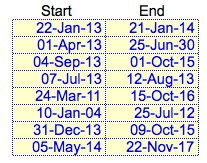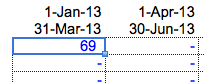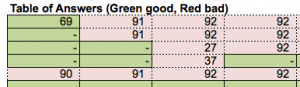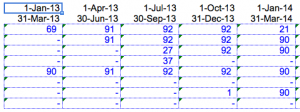# Excel Formula Challenge – Question #1 from Modeloff 2013 Released (with explanation)

If you read our last blog post, you’ll know we had the privilege of attending the Modeloff 2013 World Financial Modeling awards ceremony in NYC a few weeks ago. During the ceremony, the finalists were organized into teams by region to solve Excel formula building questions competing head-to-head for group prize money and bragging rights. Dan Mayoh from the Modeloff question team provided us with the four questions and corresponding answers, so we will discuss these questions over our next four blog posts! Here’s your chance to test your Excel skills and experience the deathmatch environment of the group challenges pictured below:## Ground Rules of Group Challenge

The goal of the challenge is to write the most “efficient” Excel formula that solves the problem, e.g. the formula with the least number of characters. The teams were generally given 10 minutes or less to create their final formulas. The formula must be copied across all the cells in the answer range to solve the objective. No VBA, Defined Names, Helper Cells, or references to the Table of Answers or Check Sum cells may be used.

## The Challenge

You have a bunch of empty cells as shown below with these start dates and end dates above them:You also have these Start and End dates in yellow directly to the left of those blue outlined cells:The Objective: Write a formula that counts the number of days between the Start and End dates in the above table that fall within the Start and End dates listed above the first table.

For instance, in the first cell in the blue-outlined table, the number 69 should be the output, like this:Why is that first cell 69? The period in the blue-outlined table is January 1st to March 31st, 2013 (one quarter). The Start date in the yellow table is January 22nd, 2013, which is greater than the period start date (January 1st, 2013). The End date from the yellow table, however, is greater than the period end date at January 21st, 2014. Therefore, the only overlap would be from January 22nd to March 31st 2013 in this case (69 days total).

The correct number in the second column for the blue-outlined table is 91. Why? The period start and end date for this cell is April 1st, 2013 and June 30th, 2013. The Start and End dates in the yellow table are April 1st, 2013 and June 25, 2030. This entire date range of 17 years overlaps with the entire period from the blue-outlined table, so the resulting answer is the entire quarter, or 91 days. If there is no overlap, then the formula should return nothing in the blue-outlined cells.

The Table of Answers shown in the Excel file tells you if the output in the blue-outlined cells are indeed the correct calculation (Green = correct, Red= incorrect):The Table of Answers quickly helps you figure out if the formula you are copying across and down in the blue-outlined table are correct. After creating the correct formula and copying it across all the cells in the range, your table should look like this:Give it a shot! Download this file that represents the data discussed above and see if you can create the formula!

## The Solution

The very first thought that comes to my mind is to use a bunch of IF statements to solve the problem. While this brute force method will work, it’s not the most efficient solution since the challenge calls for writing the shortest formula.

The solution involves using the MAX() and MIN()  formulas in a creative way. The final formula only uses 38 characters as reported by Dan:

`MAX(,MIN(H\$17,\$F18)-MAX(H\$16,\$E18)+1)`

This is the formula you enter in the first cell of the blue-outlined cells and then copy over to all the cells in the range.

## The Explanation

Why does this work? Let’s first focus on the MIN(H\$17,\$F18) part of the formula. MIN() simply returns the smallest value you provide the function—in this case it simply returns H\$17 or \$F18 depending on which is smaller. H\$17 refers to March 31st, 2013 in the blue-outlined cells, and \$F18 refers to January 21st, 2014 in the yellow cells. Ok, so we know that this MIN() formula will return March 31st, 2014 (cell H\$17).

For the MAX(H\$16,\$E18) function, MAX() does the opposite of MIN(), and returns the greater value of these two inputs. H\$16 refers to January 1st, 2013, and \$E18 refers to January 22nd, 2013.  The greater of these two dates is, of course, January 22nd, 2013.

Now, let’s step back and look at what this does: MIN(H\$17,\$F18)-MAX(H\$16,\$E18)+1

We are taking March 31st, 2014 and subtracting from it January 22nd, 2013. The result is 69 days. The reason we add the 1 at the end is to make sure the result is inclusive of the start and end dates.

If we simply copy this formula MIN(H\$17,\$F18)-MAX(H\$16,\$E18)+1 across the range of blue-outlined cells, we’ll get something like this:This is obviously incorrect since there negative numbers in parentheses that doesn’t make sense if we are trying to find the overlap. These negative numbers should in fact be empty cells since the dates from the yellow table to not overlap with the period dates from the blue-outlined table.

Here is where the MAX() function from the outside of the formula we found comes into play: MAX(,MIN(H\$17,\$F18)-MAX(H\$16,\$E18)+1)

We are asking Excel to give us the greater of MIN(H\$17,\$F18)-MAX(H\$16,\$E18)+1 or 0. If there is indeed an overlap, then we will always get a positive number from the MIN(H\$17,\$F18)-MAX(H\$16,\$E18)+1 part of the formula. When this formula returns a negative number, 0 will be the result of this outside MAX() function, so therefore the resulting output will be the 0 or the blank we are looking for in the answer set.

Interestingly, the formula could have been written like this: MAX(0,MIN(H\$17,\$F18)-MAX(H\$16,\$E18)+1).

You see that extra 0 (zero)? That can simply be deleted and Excel automatically knows to compare against the number 0 as stated in the final solution. This also allows us to save one character from formula!

## Conclusion

This is the first question the teams answered and most teams finished it in less than 10 minutes. How long did it take for you? Were you able to come up with a shorter formula?